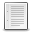File : Details

# partfrac()

Partial fraction decomposition of a rational in the C set
Details
Version
1.2
A more recent valid version with binaries for Scilab exists: 1.3.1
Author
Samuel Gougeon
Maintainer
Samuel Gougeon
Category
Supported Scilab Version
6.0
Creation Date
November 1, 2018Description
```
--> partfrac

Partial fraction decomposition of a rational in C

SYNTAX
------
partfrac    // Displays this help
I          = partfrac(r)
[I, R]      = partfrac(r)
[I, R, F]   = partfrac(r)
[I, R, F, T]= partfrac(r)

PARAMETERS
----------
r : Single rational = polynomial fraction with real or complex coefficients.
I : Integer part = non-fractional part of f (single polynomial).
R : Remainder of r: rational with degree(numerator) < degree(denominator)

F : (3xN) matrix describing the N terms of the r's decomposition:
F(1,:): real or complex coefficient of numerators.
F(2,:): real or complex value of the considered poles.
F(3,:): integer>0: powers m of the denominators (x-pole)^m
F is such that sum( F(1,:)./ ((x-F(2,:)).^F(3,:)) ) - r == 0.

T : 3-rows column of Texts. write(%io(2),t) displays i+d in a comprehensive
way. The format of coefficients is set with format().

DESCRIPTION
-----------
From a single polynomial fraction r = p/q with coprime polynomials p and q,
partfrac(f) extracts
* the non fractional part I of f: polynomial such that
0 <= degree(I) <= degree(p) - degree(q): I = p - modulo(p,q)
* the fractional part of r, or remainder R of p/q : R = modulo(p,q)/q
such that degree(r.num))< degree(q) and  r = I + R
* the rational decomposition of R, aka partial fraction decomposition of r:
The 'vector' of elementary rationals c/(x-pole)^m where c and poles are
decimal or complex numbers and m are the multiplicities of poles:
c = F(1,:), pole = F(2,:), m = d(3,:)

In addition, partfrac() may return as text the literal expression T of the
whole decomposition of r. This form shows the factorized forms of denominators
where poles that are multiple appear with their powers. write(%io(2), T) may
be used to display it.

DEPENDENCY: If F or/and T is expected, polyroots() is required (=>See also)

NOTE: pfss(r) yields a list mixing the non-fractional part (as last element)
with the decomposition, and where terms of the decomposition are not always
elementary: their denominator may be of order 2 even for real poles. See the
example.

REFERENCE
---------
Comments, scoring and bug reports are welcome on
http://fileexchange.scilab.org/toolboxes/451000#new_comment

--------
pfss      : Partial (uncomplete) fraction decomposition of a linear system
modulo    : Remainder after polynomial division
polyroots : Multiplicities and values of polynomial multiple roots:
http://fileexchange.scilab.org/toolboxes/362000
pdiv_inc  : Polynomial division with increasing terms powers:
http://fileexchange.scilab.org/toolboxes/449000

EXAMPLE
-------
x = poly(0,"x");
r = (3-x+x^2-4*x^3+2*x^4) / ((x-1)*(x-2)^2)
[I, R, F, T] = partfrac(r);
I, R, F, T
D = F(1,:)./((x-F(2,:)).^F(3,:))
clean(R-sum(D))
// Comparison with pfss():
list2vec(pfss(r)).'

RESULTS
-------

-->x = poly(0,"x");

--> r = (3-x+x^2-4*x^3+2*x^4) / ((x-1)*(x-2)^2)
r  =
2    3    4
3 - x + x - 4x + 2x
-------------------
2   3
- 4 + 8x - 5x + x

--> [I, R, F, T] = partfrac(r);

--> I,R,F,T
I  =
6 + 2x

R  =
2
27 - 41x + 15x
--------------
2   3
- 4 + 8x - 5x + x

F  =
14.    5.    1.
2.     2.    1.
1.     2.    1.

T  =
!          14       5        1    !
!6 + 2x + ---- + -------- + ----  !
!         -2+x   (-2+x)^2   -1+x  !

--> D = F(1,:)./((x-F(2,:)).^F(3,:))

D  =
!     14            5             1     !
!  ---------  --------------  --------- !
!                          2            !
!  -2 +  1x    4 - 4x +  1x   -1 +  1x  !

--> clean(R-sum(D))
ans  =
0
-
1

--> // Comparison with pfss():

--> list2vec(pfss(f)).'
ans  =

- 23 + 14x        1       6 + 2x
----------     -----     ------
2
4 - 4x + x   - 1 + x       1
```Files (1)OS-independent binary for Scilab .x
```File defining the function partfrac(), help contents and examples

* MAKE partfrac() AVAILABLE in EVERY SESSION: Click here to see HOW TO

* Get help: Enter "partfrac" without parameters

Note: The FE admin has reversionned all Files sets.
For partfrac(): the version 2.0 is now the version 1.1.
Then:

CHANGES 1.1 => 1.2
------------------
* denom() and numer() replaced (deprecated in Scilab 6.0)
* Bug fixed when the integer part I is a scalar.
* Help: Inconsistent notations fixed.

```News (0)Comments (1)Comment from Man Jane -- August 4, 2020, 10:54:12 PM
```https://primusrewe.me/login/
```